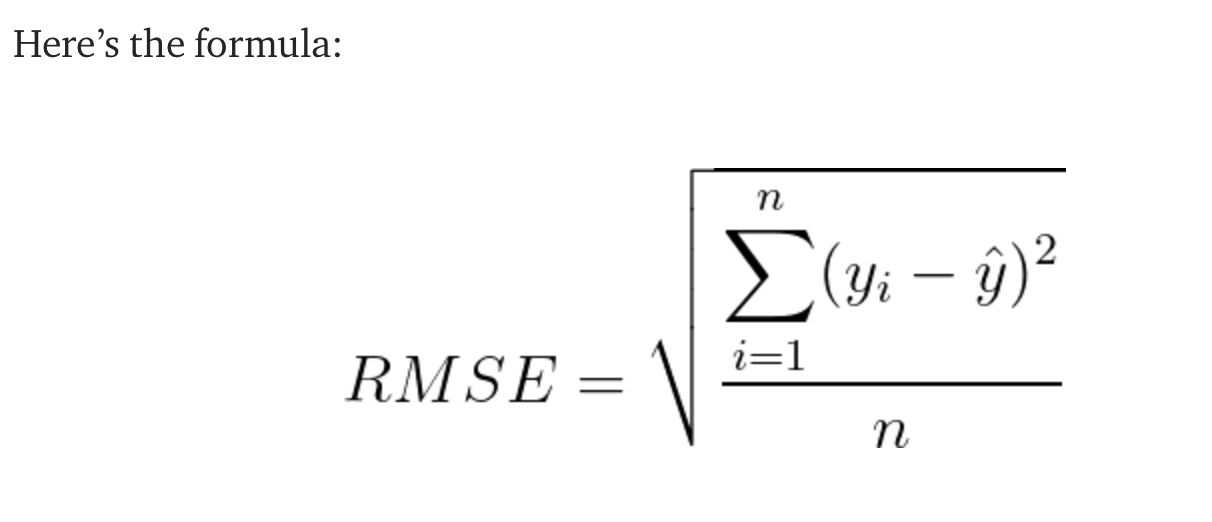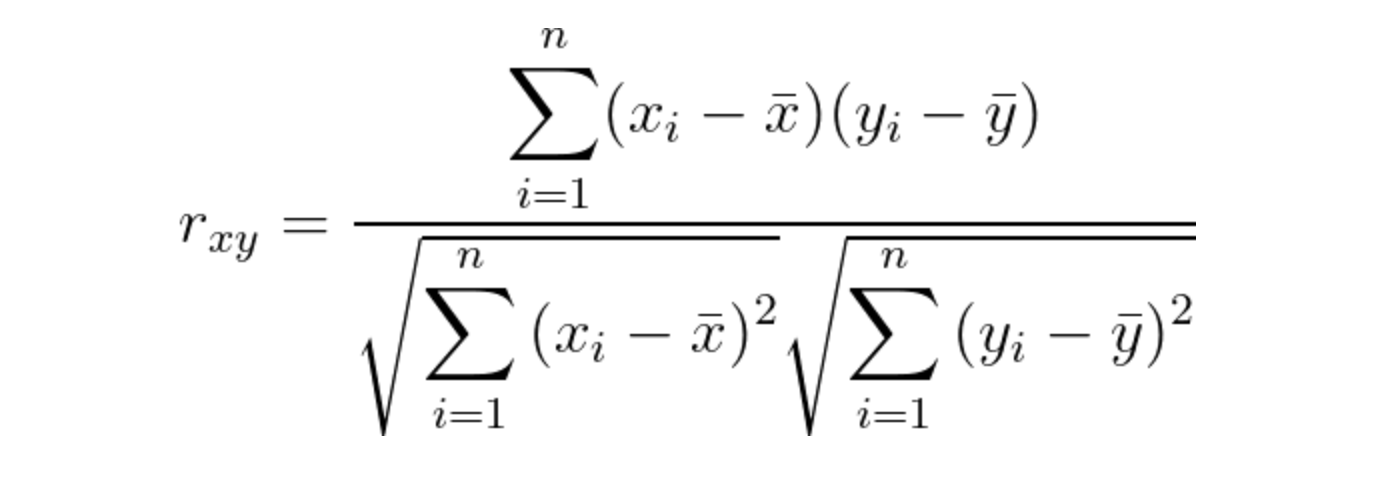## Acronyms

• DOF: Degrees-of-Freedom
• DOA: Degrees of actuation of a prosthesis.

So what is the difference 😀?

DoAs represent the number of independent actuator/motor, while DoF represent the number of movements we can execute on the prosthetic hand with these motors. For example, if a prosthetic hand has 5 intrinsic motors to control flexing and extension of the five fingers then we have 10 DOFs and 5 DOAs.

## Common concepts and ideas

• Continuous control is also often refered to as proportional control. This paper by Fougner et al., 2012 provide a really nice overview on terminology and literature related to proportional control. Quoting from the paper:

Proportional control is exhibited by a prosthesis system if and only if the user can control at least one mechanical output quantity of the prosthesis (e.g. force, velocity, position or any function thereof) within a finite, useful, and essentially continuous interval by varying his/her control input within a corresponding continuous interval.

• Given this definition, proportional control does not necessarily mean that relationship between the input and output of the system are proportional in a strict mathematical sense. It just means that changes in the EMG signal (i.e input to the system) lead to changes in the motor output of the system. This is to be contrasted with the on-off control in which there are only two possible outputs of the system (i.e turning on or off a prosthetic function).
• A simple example of proportional control as extracted, again, from Fougner et al., 2012:

“…a system in which the electromyogram (EMG) from flexors and extensors of the user’s forearm is measured, amplified, filtered and smoothed by two active electrodes. This provides estimates of EMG amplitudes that can be sent to a hand controller. After applying thresholds to remove uncertainty at low contraction levels, the controller sets a voltage applied to the motor that is proportional to the contraction intensity”.

• People often talk about intuitiveness of a decoder or control strategy. What they mean by that is how natural the control scheme is or put differently how similar the motor functionality provided by the system is to that of a biological limb. It follows that the more proportional the control is the more intuitive it is. Intuitive controllers are the ones that map patterns of muscle co-activations to prosthesis DOAs. Therefore, we get a wider range of class of motions.

## Regression-based control

### Models used

I kept coming back to these models when reading about regression-based control:

• Kalman filter
• Wiener filter
• Linear and non-linear regression algorithm (e.g. ANN, Random Forest Regression,…)

#### Wiener Filter

Without going into a lot of mathematical details (because I would have to revise some mathematical foundations to be able to grasp everything), what I know for now is that:

• A Wiener filter is a signal processing technique for estimating a target variable using linear time-invariant filtering applied to a measured noisy signal. Speaking of “filters” usually lead to a discussion about convolution :D. In fact, the Wiener filter operation is about convolving the input signal (in my case this is EMG feature) with a finite impulse response function to produce an output y (e.g. position of a single DOA of the prosthetic hand).

#### Linear Regression

• To evaluate a linear regression model, often $R^2$ (Coefficient of determination which is the square of the correlation coefficient R) and the RMSE (Root Mean Squared Error).
• In RMSE, the difference between predicted and actual (called residuals). To compute the RMSE, we average the squared residuals then take the square root to put the metric back in the response variable ($y$) scale.extracted from this article

• $R^2$ measures the strength of the relationship between the response and the predictor variables in the model. Higher $R^2$, then means that the predictor variable is characterizing the variance observed in the response variable. Put differently, $R^2$ shows how well the variation we see in $y$ can be explained by the input $x$$R^2$ formula

Krasoulis, Agamemnon, Sethu Vijayakumar, and Kianoush Nazarpour. “Effect of User Practice on Prosthetic Finger Control With an Intuitive Myoelectric Decoder.” Frontiers in Neuroscience 13 (2019). I might do a more extensive summary of the paper later.

### Aim of this paper

To study the effect of user adaptation in continuous finger position control. The idea is that it was previously shown on classification tasks that user adaptation plays a huge role in improving the control accuracy. Here they extend this to continuous control where they hypothesize that:

1. During closed-loop interaction with the prosthesis can improve the intuitive decoder, one this is mapping EMG features to prosthetic digit positions
2. there is a need to evaluate the trained models in online setting and that offline decoding do not reliably predict real-time control outcomes

### Decoder model

• They use Wiener filter to decode finger positions form sEMG, (Ninapro DB8). The model performance is evaluated offline.
• A real-time control mode was also tested in which the trained model was used